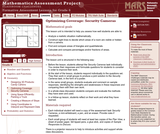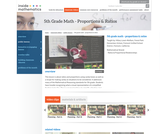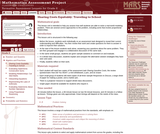Updating search results...

# 4 Results

View
Selected filters:
• WY.Math.6.RP.A.1Conditional Remix & Share Permitted
CC BY-NC-SA
Rating
0,0 stars

In this activity, learners use their hands as tools for indirect measurement. Learners explore how to use ratios to calculate the approximate height of something that can't be measured directly by first measuring something that can be directly measured. This activity can also be used to explain how scientists use indirect measurement to determine distances between things in the universe that are too far away, too large or too small to measure directly (i.e. diameter of the moon or number of bacteria in a volume of liquid).

الموضوع:
الرياضيات
نوع المادة:
Activity/Lab
Provider:
متحف Exploratorium لدعم التعليم
المؤلف:
Exploratorium
Gordon and Betty Moore Foundation
National Science Foundation
The Exploratorium
12/07/2010Only Sharing Permitted
CC BY-NC-ND
Rating
0,0 stars

This lesson unit is intended to help sixth grade teachers assess how well students are able to: Analyze a realistic situation mathematically; construct sight lines to decide which areas of a room are visible or hidden from a camera; find and compare areas of triangles and quadrilaterals; and calculate and compare percentages and/or fractions of areas.

الموضوع:
هندسة الشكل
Ratios and Proportions
نوع المادة:
التقييم
Lesson Plan
Provider:
Shell Center for Mathematical Education
Provider Set:
Mathematics Assessment Project (MAP)
04/26/2013قراءة النص المطبوع بخط رفيع
Rating
0,0 stars

This lesson is about ratios and proportions using candy boxes as well as a recipe for making candy as situations to be considered. It addresses many Mathematical Reasoning standards and asks students to: Use models to understand fractions and to solve ratio problems; think about a ratio as part/part model and to think about the pattern growing in equal groups or a unit composed of the sum of the parts; find a scale factor and apply it to a ratio. (5th Grade Math)

الموضوع:
Ratios and Proportions
نوع المادة:
Activity/Lab
Lecture
Lesson Plan
Teaching/Learning Strategy
Provider:
Noyce Foundation
Provider Set:
في قلب الرياضيات
المؤلف:
Lewis-Wolfsen, Hillary
11/30/2011Only Sharing Permitted
CC BY-NC-ND
Rating
4,0 stars

This lesson unit is intended to help teachers assess how well students are able to solve a real-world modeling problem. There are several correct approaches to the problem, including some that involve proportional relationships.

الموضوع:
Ratios and Proportions
نوع المادة:
التقييم
Lesson Plan
Provider:
Shell Center for Mathematical Education
Provider Set:
Mathematics Assessment Project (MAP)